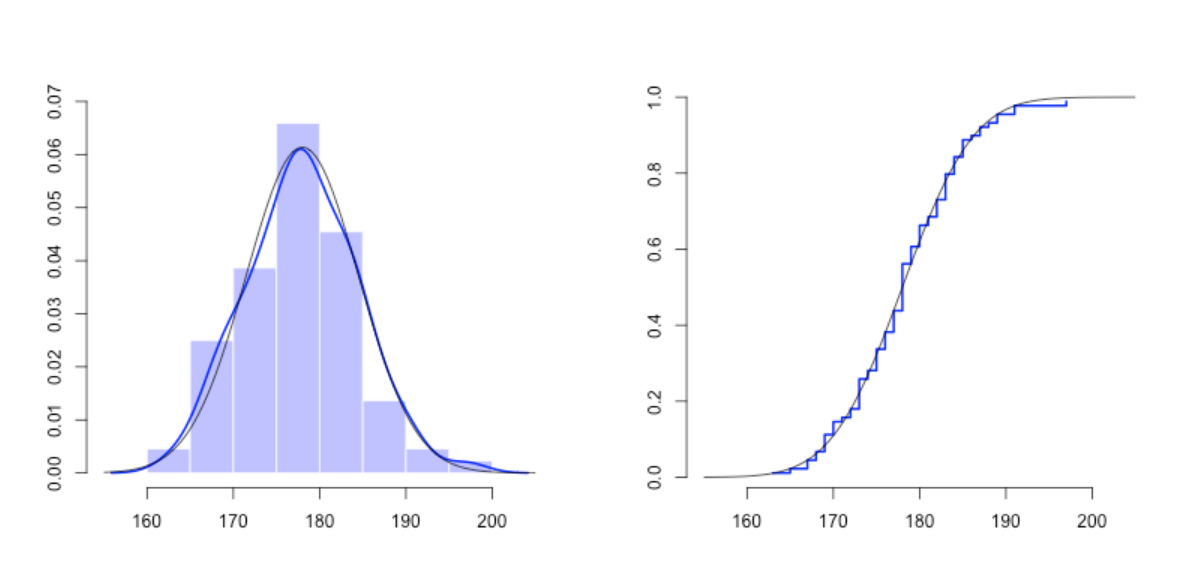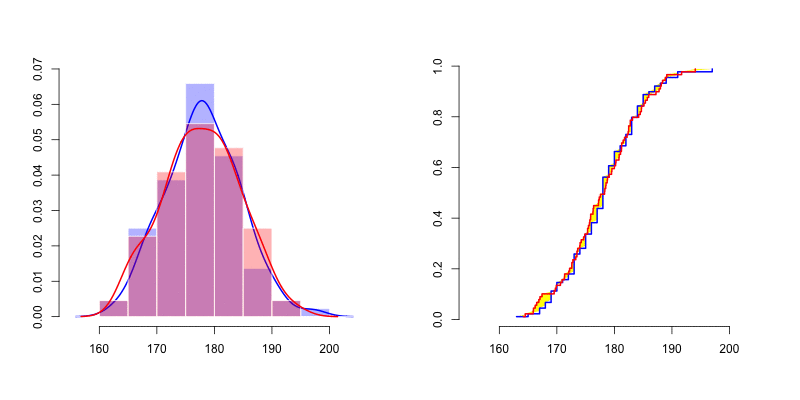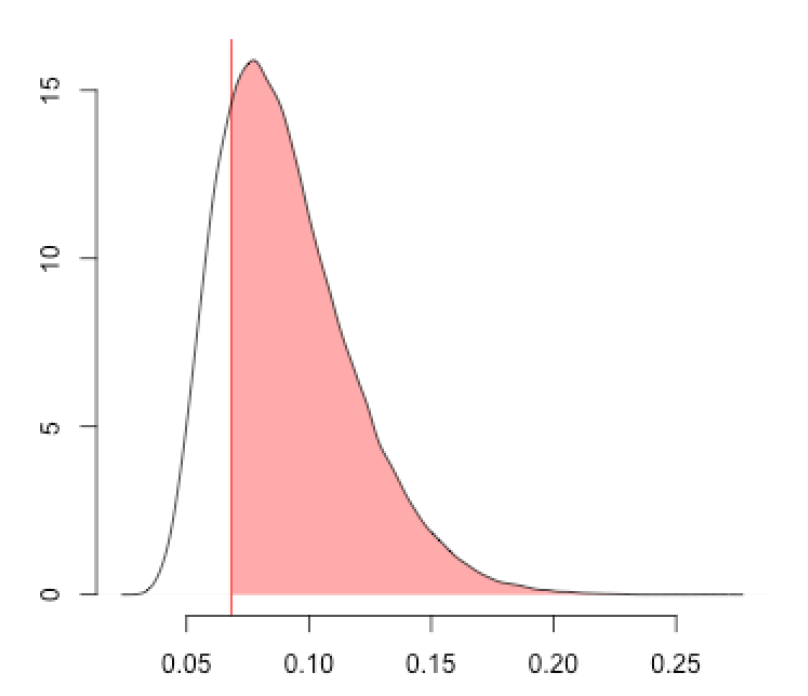# 原文链接：### 如何使用蒙特卡洛模拟来推导随机变量可能的分布，我们回到统计数据（无协变量）进行说明。我们假设观察值是基础随机变量，具有未知分布的随机变量。

`X=Davis\$height[Davis\$sex=="M"]`

`u=seq(155,205,by=.5)par(mfrow=c(1,2))hist(X,col=rgb(0,0,1,.3))lines(density(X),col="blue",lwd=2)lines(u,dnorm(u,178,6.5),col="black")Xs=sort(X)n=length(X)p=(1:n)/(n+1)plot(Xs,p,type="s",col="blue")lines(u,pnorm(u,178,6.5),col="black")``mean(dks) 0.78248`

`ks.test(X,"pnorm",178,6.5)	One-sample Kolmogorov-Smirnov testdata:  XD = 0.068182, p-value = 0.8079alternative hypothesis: two-sided`

1.

2.

3.

5.

6.

7.

8.

9.

• 博文量
87
• 访问量
38573